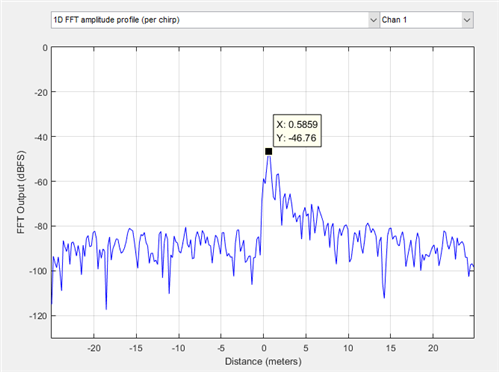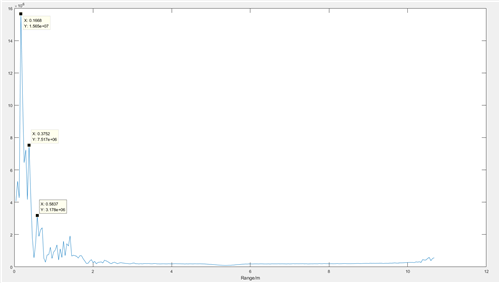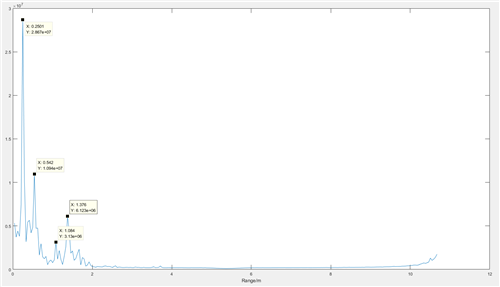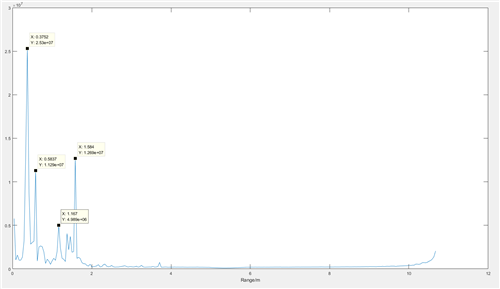# AWR1443：处理静态对象数据存在问题

你好，Fig.1 range=0.5mFig.2 range=1mFig.2 range=1.5m

M1=fft(a);
a1=sum(abs(M1),2);
[m1,m2]=size(a1);
t=1:256;
plot(t*delta,a1);
xlabel('Range/m');